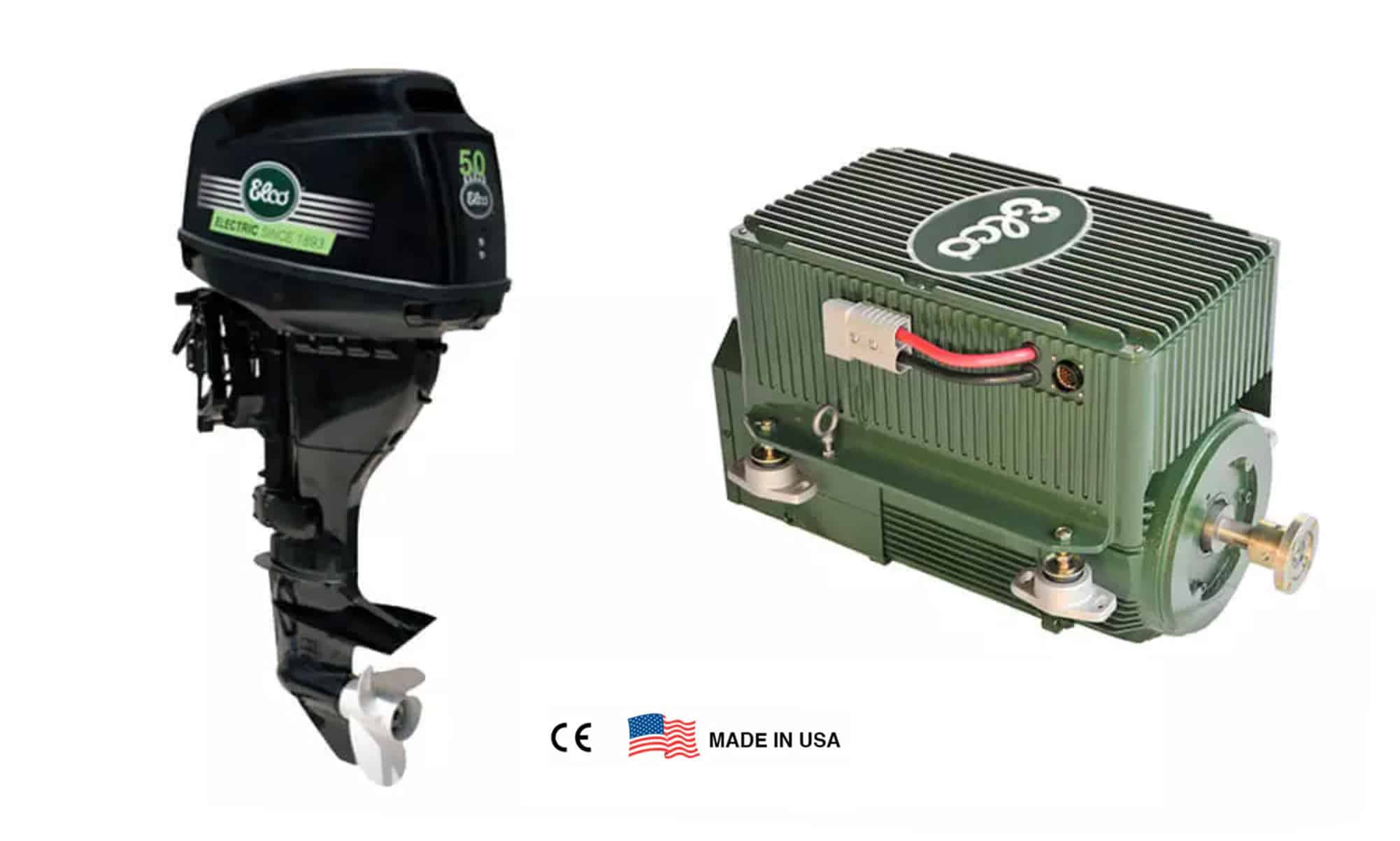### 1. What are the benefits of repowering?

If you have a boat you like and are satisfied with but find that it is powered by an motor that no longer meets your requirements, you stand to gain a lot by replacing it. Thanks to the fast pace of technological developments in recent years, you are going to notice some major benefits when you change to a new drive system:

• Increased safety and reliability
• Improved comfort
• Better cruising pleasure and more secure maneuvering
• Higher performance
• Immensely reduced environmental impact
• Easier adaptation to modern accessories
• Lower operating cost
• Increased value for your boat

### 2. What are the benefits of electric power?

• No exhaust fumes produced
• Silent motor operation – No noise pollution
• Very small carbon footprint
• Cruise all day and charge the batteries overnight for just pennies
• Never needs a tune-up; no need to winterize

### 3. What is electric power?

Power = volts x amperes

The electric power in watts associated with a complete electric circuit. A circuit represents the rate at which energy is converted from the electrical energy of the moving charges to some other form, e.g. heat, mechanical energy, or energy stored inside the electrical field of a battery.

### 4. How do you convert watts (W) to kilowatts (kW)?

kW = W / 1000

The power in kilowatts is equal to the power in watts divided by 1000.
Example: Convert 1200 watts to kilowatts
1200 W / 1000 kW = 1.2 kW

### 5. How do you convert kilowatts (kW) to horsepower (hp)?

One horsepower is equal to .745699872 kilowatts
Example: Convert 1.2 kW to horsepower
1.2 kW / .746 = 1.609 hp

### 6. How do you convert watts to amps?

A = W / V

The current in amps is equal to the power in watts divided by voltage in volts.
Example: 4000 watts and 48 volts is how many amps?
4000 / 48 = 83.33 amps

### 7. If I produce 83 amps and 48 volts, how much power do I have?

W = A x V

Watts  = amps x volts
83 x 48 = 3,984 watts = 3.984 kW (3984/1000)

### 8. If I produce 3.984 kilowatts (kW), how much horsepower (hp) does that equal?

hp ≈ kW / 0.746

1hp = .745699872 kilowatts
3.984 kW / .746 = 5.34 hp

### 9. What is the Bollard Pull Test?

Bollard pull is a measurement of the pulling power of a watercraft. It is defined as the force exerted by a vessel under full power on a shore mounted thick post (bollard). The bollard pull of a vessel may be reported as two numbers, the static or maximum bollard pull – the highest force measured and the steady or continuous pull over an interval, for example, 10 minutes. Bollard pull is primarily used for measuring the strength of tugboats.

### 10. How fast can I go?

Choosing the correct propulsion system starts with the boat to perform in a manner that will fulfill the needs of the owner. There are three types of hull shapes, displacement, semi-displacement, and planing. Displacement (deep V, sailboat, kayak, etc.) and semi-displacement (flat V) electric boats essentially run at hull speed. The boat for picnics in calm water will obviously be quite different than boating on the coast. However, displacement and semi-displacement electric boats essentially run at hull speed. Hull speed is equal to 1.34 x square root of the load waterline length (LWL). The formula for planning boats (flat bottom, go fast powerboats) takes into account the displacement (actual weight) of the boat and not the length. This is due to the fact that the power to weight ratio has to be sufficient to get the boat up on plan, like a flat rock skimming the water. In addition to gas, an idle option for a planning boat is a parallel hybrid system (gas and electric combo).

Load waterline length (LWL) of boat Hull speed (knots)
9 feet 4.02
18 feet 5.7
22 feet 6.3
30 feet 7.3

### 11. How many batteries do I need for my electric outboard?

The 5, 7, and 9.9 electric outboards are all 48 volt systems. If you select a 12 volt lead acid AGM battery, you will need 4 batteries. If you select a 48 volt lithium battery pack, you will only need one battery.

### 12. How far can I go?

Ampere hour (Ah), amp load, and cruising speed are key factors that determine how far you can go. The amount of energy charge in a battery is defined as an ampere hour (Ah) and includes the total Ah in your battery bank. Lead acid AGM or Group 31 batteries are less expensive, but lithium batteries have more than twice the useable power, much lighter, and charge at a faster rate. Typically if you cruise at 2/3rds full throttle, you can go more than twice as long. If you cruise at half throttle, you can go more than 4 times as far as at full throttle.

### 13. How do I calculate expected runtimes?

Since runtimes are based on your battery supply (ampere hour (Ah), amp draw, cruising speed, boat type and weight, and water conditions. Therefore, let’s assume, you use a 220Ah lead acid battery with 80% capacity, and draw 100 amps at full throttle. Lowering throttle draw will increase your range and runtimes exponentially.Example: Run at a low throttle (about 3.5 knots, boat and conditions dependent)

Step 1: Ampere hour (Ah): 220 Ah
Step 2: 220 Ah x .80 (capacity rate) = 176Ah
Step 3: 100 amps / 4 = 25 amps (low throttle)
Step 4: 176 Ah / 25 amps = 7.04 hours of run time

### 14. How long does it take to charge my batteries?

The key factors that determine recharge time are the capacity of your batteries (ampere hour), charge rate (charger amps), depth of discharge (DOD), and efficiency loss.